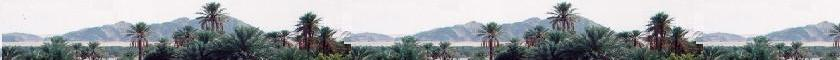##### Contents

A sigle wave

Superposition of waves

© The scientific sentence. 2010

# Meaning of the wave function

### 1.Intensity of the electromagnetic wave

Let's recall that the power of a wave is the rate at which its energy is propagated that is P = U/Δt Where U is the total energy of the wave passing through Δx during the time Δt. The quantity that characterizes the flow of energy is called intensity, that is the power of the propagating wave per unit area of a surface ΔA.
This intensity is then written as I = P/ΔA(in watts per square meters: W/m2).
We have: I = U /Δt/ΔA

For electromagntic waves, the total (electric and magnetic) energy per volume unit, that is the energy density, is expressed as: uem = εo E2; where E is the electric field of the electromagnetic wave, that can be expressed as: E = Eo sin (ωt - kx).

The related intensity is S = U /Δt/ΔA = uem ΔV/Δt/ΔA, where ΔV is the volume unit around Δx during the time Δt. Using ΔV = ΔA Δx, we can write S = uem Δx/Δt. The intensity S is the magnitude of the Pointing vector of the the wave. Further, we can write S = uem c; where c = Δx/Δt is the speed of the electromagnetic wave, that is the speed of light.
But the Pointing Vector is defined as S = E x B/μo. According to E = c B, we have: S = E2/c μo.
As εoμo c2 = 1, then: S = c εoE2. ( We have also the average S = (c εo/2)Eo2, because over the period T, the average of sin2 (ωt - kx) = 1/2 )
We have also:
I = U /Δt/ΔA = uem ΔV /Δt/ΔA = uem Δx /Δt = c uem. Where c = ΔV /ΔA = Δx , and c = Δx /Δt. Hence:

I = c εo E2. We find the expreesion of the Pointing vector.

### 2. Maining of the wave function

We remark then, the intensity of the wave is proportional to the square of the wave function. That is, if we write Ψ = E = Eo sin (ωt - kx), we can simply write I = Constant Ψ2. We can then express the total intensity as Itot = Σ I = Constant Σ Ψ2.

The probability to find a particle light in the elementary volume dV, at the time "t" is P(t) = I/Itot = Ψ2/Σ Ψ2 = Ψ2 dV/∫ Ψ2dV.
This integrale (from - ∞ to + ∞) is normalized to 1. We have then: P(t) = Ψ 2 dV. As the wave function Ψ is often complex, we write instead: P(t) = |Ψ|2 dV

P(t) = |Ψ|2 dV is the probabilty to find the particle-wave at the time t in the volume dV
|Ψ|2 is called the probability density of the wave Ψ.

This is what Max Born (1928) has set.

©: The scientificsentence.net. 2007.Web ScientificSentence

 chimie labs | scientific sentence | java | Perl | php | green cat | contact |